# Blackbody¶

A blackbody or planckian radiator is an ideal thermal radiator that absorbs completely all incident radiation, whatever the wavelength, the direction of incidence or the polarization. 

A blackbody in thermal equilibrium emits electromagnetic radiation called blackbody radiation.

## Planck's Law¶

The spectral radiance of a blackbody at thermodynamic temperature $T [K]$ in a medium having index of refraction $n$ is given by the Planck's law equation: 

$$\begin{equation} L_{e\lambda}(\lambda,T)=\cfrac{C_1n^{-2}\lambda^{-5}}{\pi}{\Biggl[\exp\biggl(\cfrac{C_2}{n\lambda T}\biggr)-1\Biggr]^{-1}} \end{equation}$$

where \begin{equation} \begin{aligned} C_1&=2\pi hc^2\\ C_2&=\cfrac{hc}{k} \end{aligned} \end{equation}

$h$ is Planck's constant, $c$ is the speed of light in vacuum, $k$ is the Boltzmann constant and $\lambda$ is the wavelength.

As per CIE 015:2004 Colorimetry, 3rd Edition recommendation $C_2$ value when used in colorimetry should be $C_2= 1,4388x10^{-2}mK$ as defined by the International Temperature Scale (ITS-90).

$C_1$ value is given by the Committee on Data for Science and Technology (CODATA) and should be $C_1=3,741771x10^{16}Wm^2$.

In the current CIE 015:2004 Colorimetry, 3rd Edition recommendation, colour temperature and correlated colour temperature are calculated with $n=1$.

Colour implements various blackbody computation related objects in the colour.colorimetry sub-package:

In :
import colour.colorimetry


Note: colour.colorimetry package public API is also available from the colour namespace.

The Planck's law is called using either the colour.planck_law or colour.blackbody_spectral_radiance definitions, they are expecting the wavelength $\lambda$ to be given in nanometers and the temperature $T$ to be given in degree kelvin:

In :
import colour

colour.colorimetry.planck_law(500 * 1e-9, 5500)

Out:
20472701909806.578

Generating the spectral distribution of a blackbody is done using the colour.sd_blackbody definition:

In :
with colour.utilities.suppress_warnings(python_warnings=True):
colour.sd_blackbody(6500, colour.SpectralShape(0, 10000, 10))


With its temperature lowering, the blackbody peak shifts to longer wavelengths while its intensity decreases:

In :
from colour.plotting import *

In :
colour_style();

In :
# Plotting various *blackbodies* spectral distributions.
blackbodies_sds = [colour.sd_blackbody(i, colour.SpectralShape(0, 10000, 10))
for i in range(1000, 15000, 1000)]
with colour.utilities.suppress_warnings(python_warnings=True):
plot_multi_sds(blackbodies_sds,
y_label='W / (sr m$^2$) / m',
use_sds_colours=True,
normalise_sds_colours=True,
legend_location='upper right',
bounding_box=[0, 1000, 0, 2.25e15]);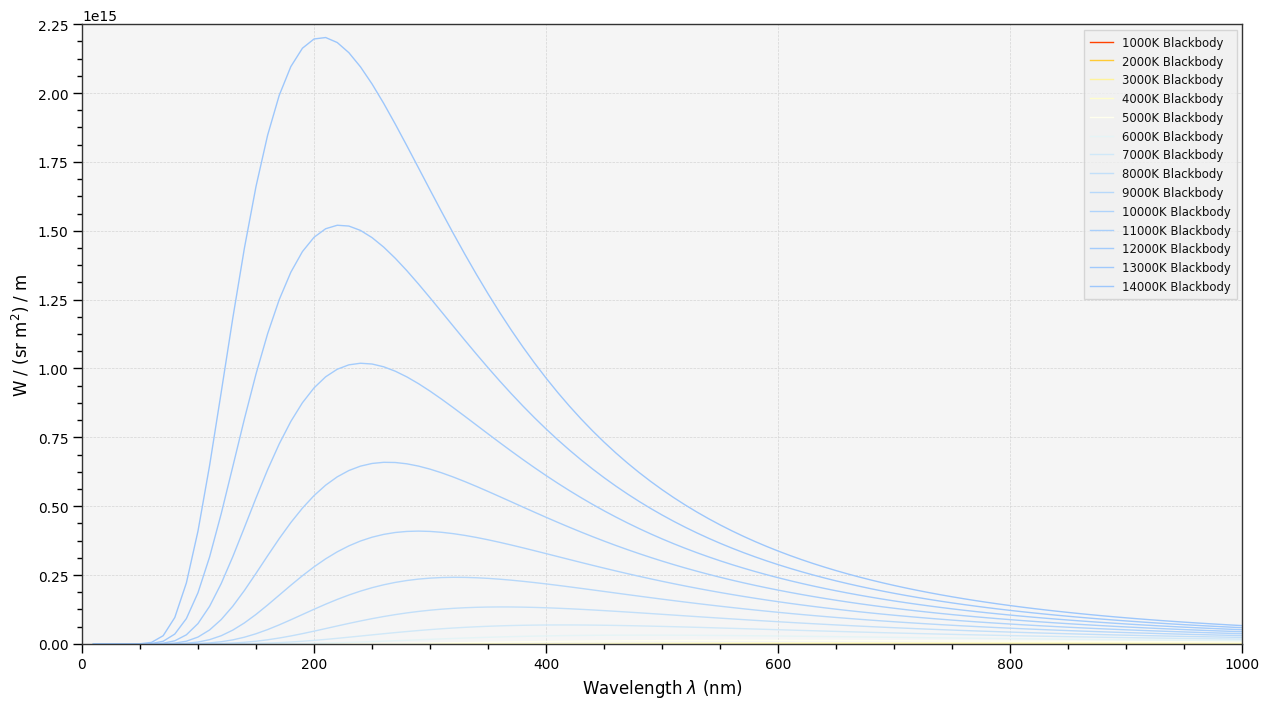Let's plot the blackbody colours from temperature in domain [150, 12500, 50]:

In :
plot_blackbody_colours(colour.SpectralShape(500, 12500, 50));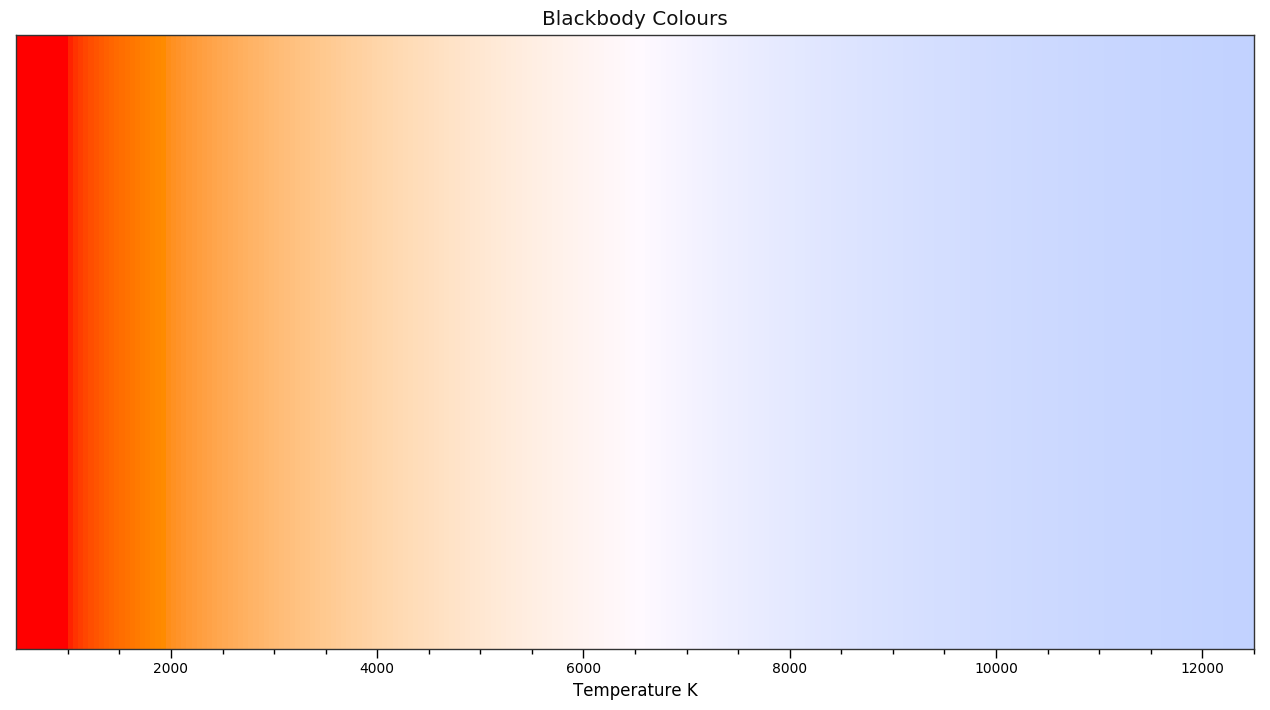## Stars Colour¶

Let's compare the extraterrestrial solar spectral irradiance to the blackbody spectral radiance of a thermal radiator with a temperature of 5778 K:

In :
# Comparing theoretical and measured *Sun* spectral distributions.
# Arbitrary ASTMG173_ETR scaling factor calculated with
# :def:colour.sd_to_XYZ definition.
ASTMG173_sd = ASTMG173_ETR.copy() * 1.37905559e+13

blackbody_sd = colour.sd_blackbody(
5778,
ASTMG173_sd.shape)
blackbody_sd.name = 'The Sun - 5778K'

plot_multi_sds([ASTMG173_sd, blackbody_sd],
y_label='W / (sr m$^2$) / m',
legend_location='upper right');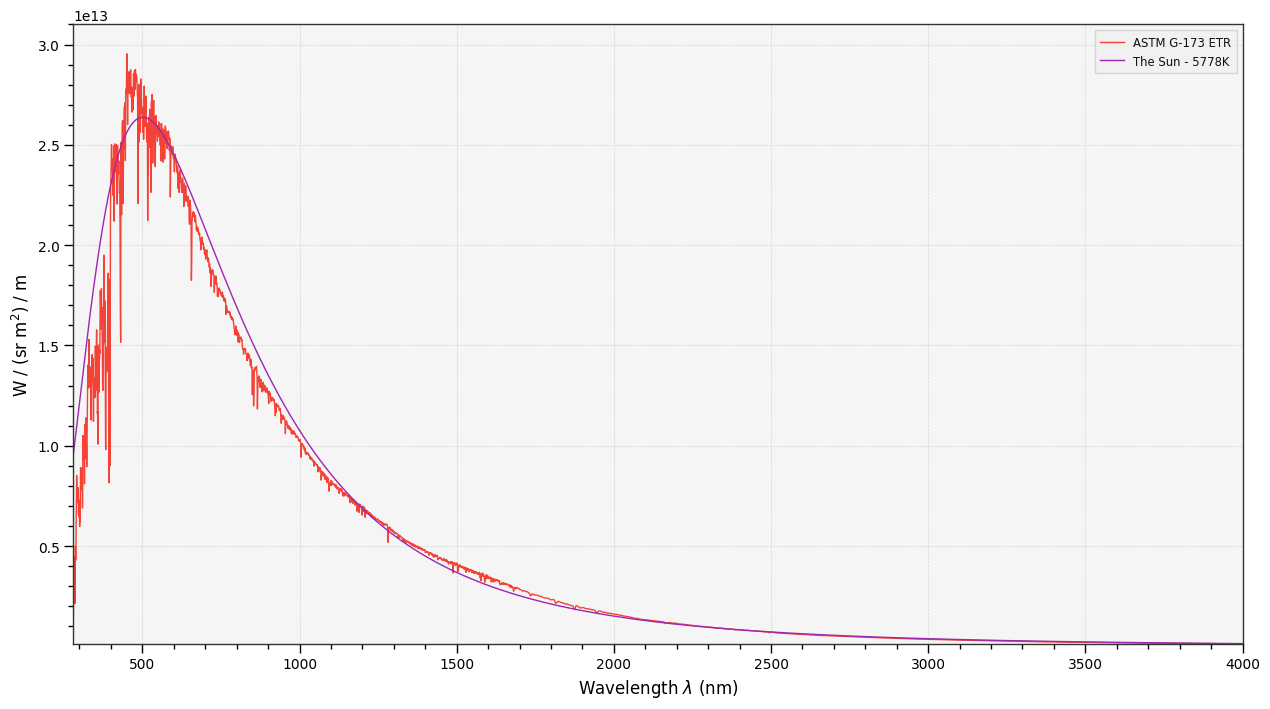As you can see the Sun spectral distribution is very close to the one from a blackbody at similar temperature $T$.

Calculating theoritical colour of any star is possible, for example the VY Canis Majoris red hypergiant in the constellation Canis Major.

In :
plot_blackbody_spectral_radiance(temperature=3500, blackbody='VY Canis Majoris');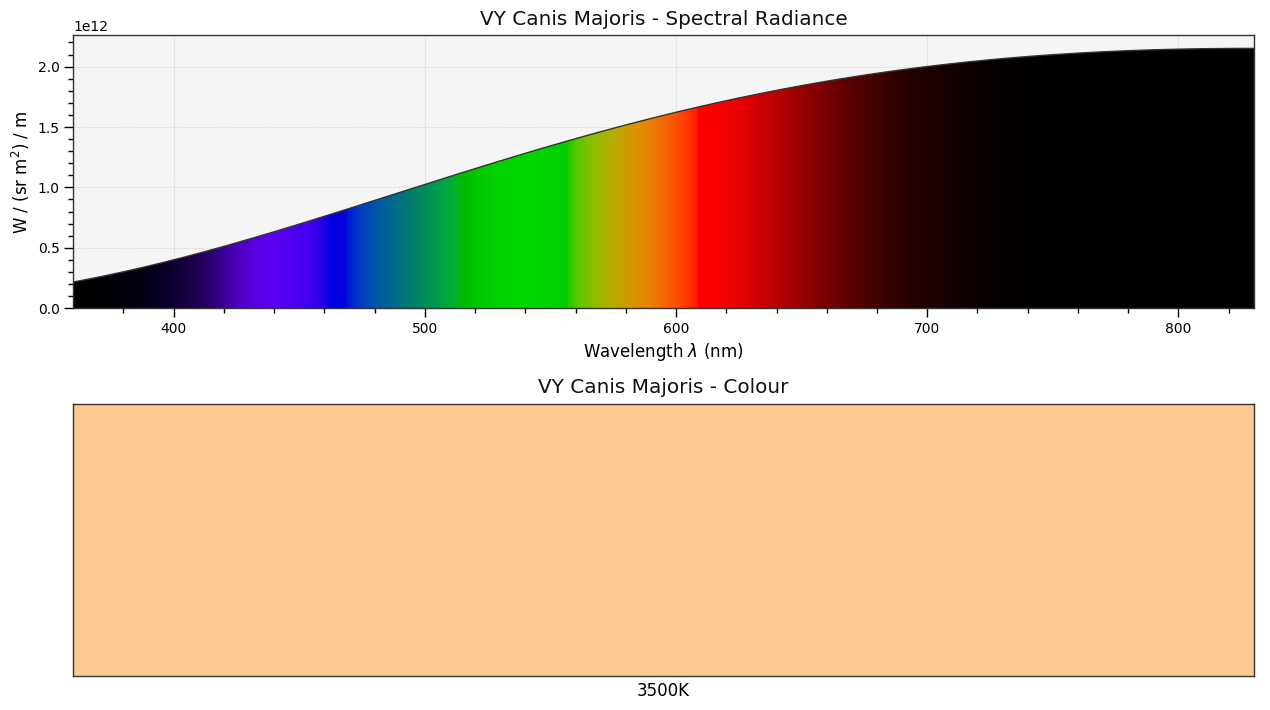Or Rigel the brightest star in the constellation Orion and the seventh brightest star in the night sky.

In :
plot_blackbody_spectral_radiance(temperature=12130, blackbody='Rigel');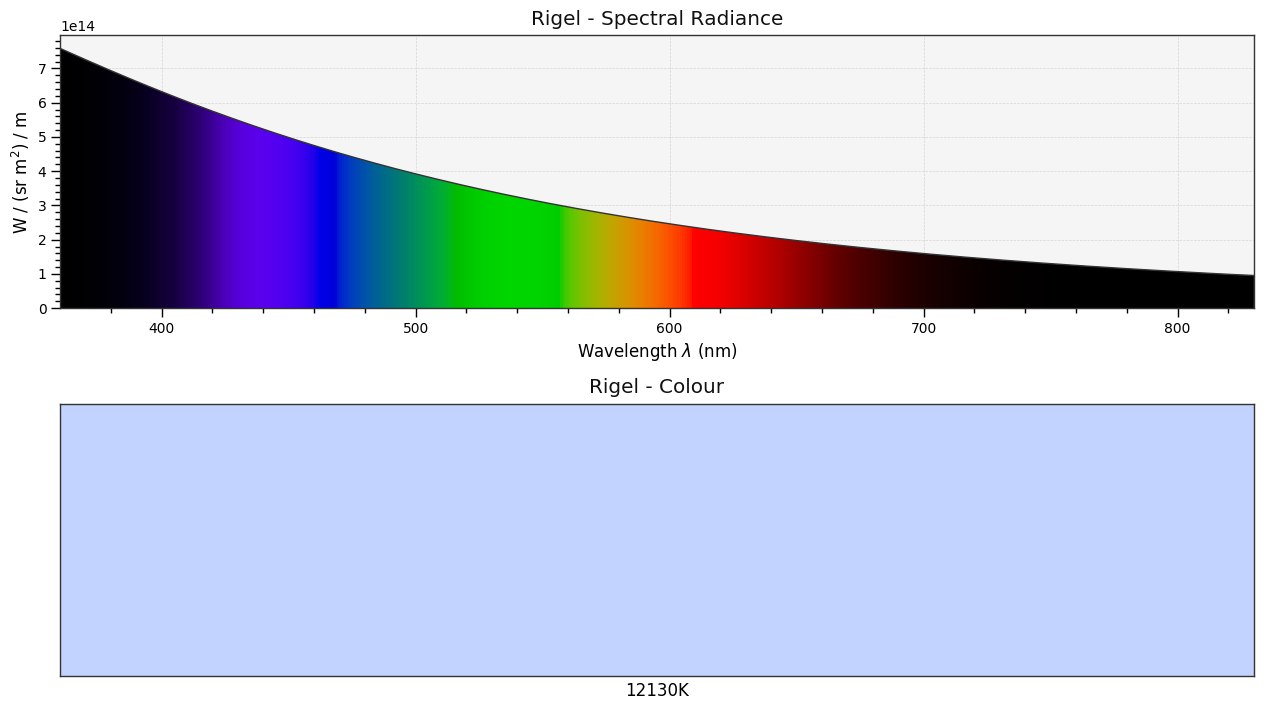And finally the Sun, our star:

In :
plot_blackbody_spectral_radiance(temperature=5778, blackbody='The Sun');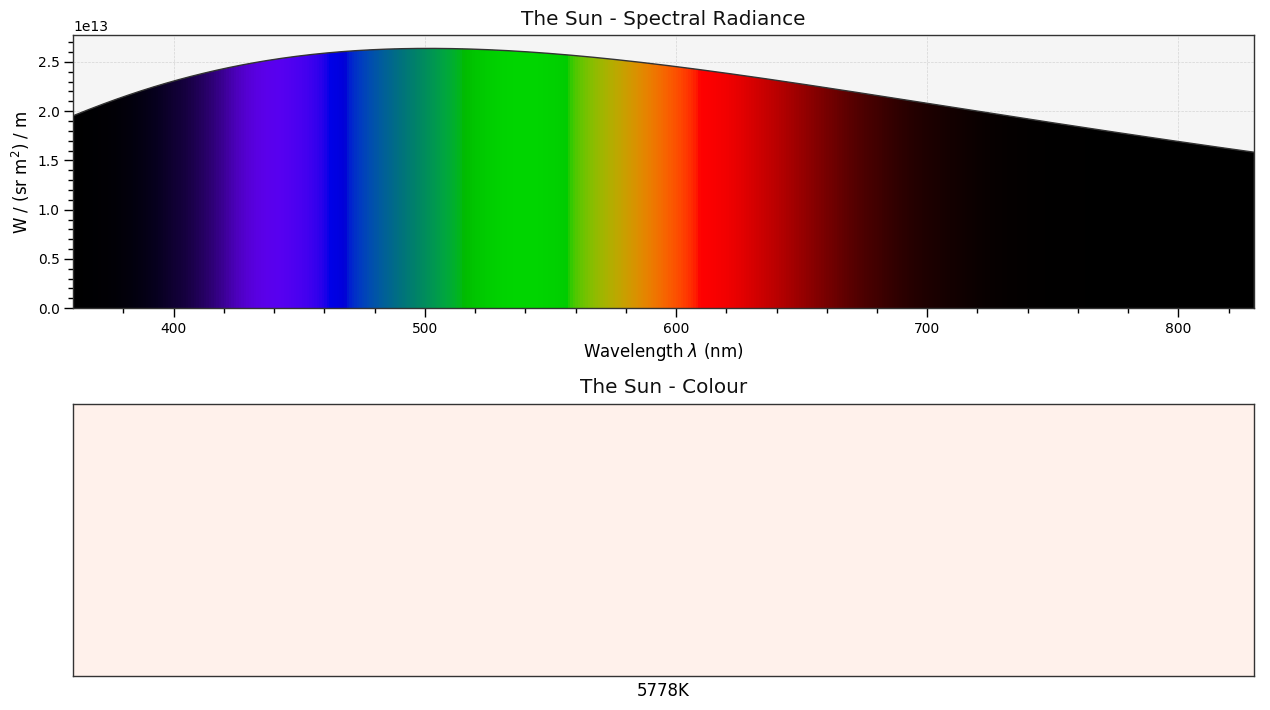## Bibliography¶

1. ^ CIE. (n.d.). 17-960 Planckian radiator. Retrieved June 26, 2014, from http://eilv.cie.co.at/term/960
2. ^ CIE TC 1-48. (2004). APPENDIX E. INFORMATION ON THE USE OF PLANCK’S EQUATION FOR STANDARD AIR. In CIE 015:2004 Colorimetry, 3rd Edition (pp. 77–82). ISBN:978-3-901-90633-6